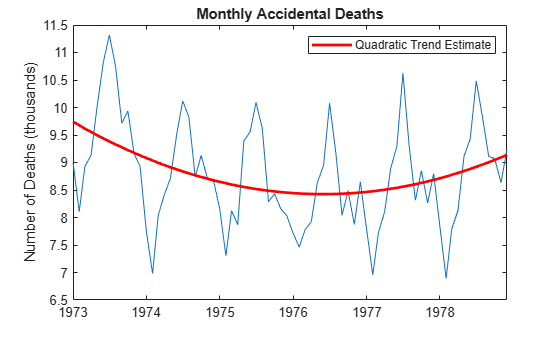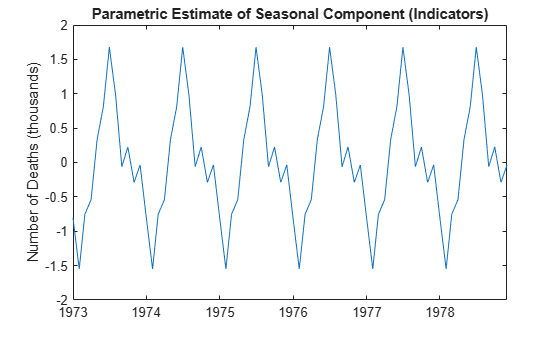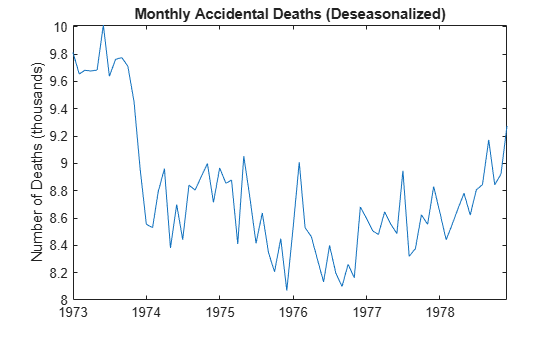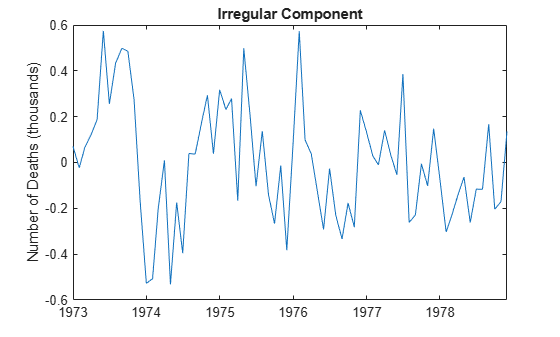Main Content

# Parametric Trend Estimation

This example shows how to estimate nonseasonal and seasonal trend components using parametric models. The time series is monthly accidental deaths in the U.S. from 1973 to 1978 (Brockwell and Davis, 2002).

### Step 1: Load the Data

Load the accidental deaths data set.

```load Data_Accidental y = Data; T = length(y); figure plot(y/1000) h1 = gca; h1.XLim = [0,T]; h1.XTick = 1:12:T; h1.XTickLabel = datestr(dates(1:12:T),10); title 'Monthly Accidental Deaths'; ylabel 'Number of Deaths (in thousands)'; hold on```The data shows a potential quadratic trend and a strong seasonal component with periodicity 12.

### Step 2: Fit Quadratic Trend

Fit the polynomial

`${T}_{t}={\beta }_{0}+{\beta }_{1}t+{\beta }_{2}{t}^{2}$`

to the observed series.

```t = (1:T)'; X = [ones(T,1) t t.^2]; b = X\y; tH = X*b; h2 = plot(tH/1000,'r','LineWidth',2); legend(h2,'Quadratic Trend Estimate') hold off```### Step 3. Detrend Original Series.

Subtract the fitted quadratic line from the original data.

`xt = y - tH;`

### Step 4. Estimate Seasonal Indicator Variables

Create indicator (dummy) variables for each month. The first indicator is equal to one for January observations, and zero otherwise. The second indicator is equal to one for February observations, and zero otherwise. A total of 12 indicator variables are created for the 12 months. Regress the detrended series against the seasonal indicators.

```mo = repmat((1:12)',6,1); sX = dummyvar(mo); bS = sX\xt; st = sX*bS; figure plot(st/1000) title 'Parametric Estimate of Seasonal Component (Indicators)'; h3 = gca; h3.XLim = [0,T]; ylabel 'Number of Deaths (in thousands)'; h3.XTick = 1:12:T; h3.XTickLabel = datestr(dates(1:12:T),10);```In this regression, all 12 seasonal indicators are included in the design matrix. To prevent collinearity, an intercept term is not included (alternatively, you can include 11 indicators and an intercept term).

### Step 5. Deseasonalize Original Series

Subtract the estimated seasonal component from the original series.

```dt = y - st; figure plot(dt/1000) title 'Monthly Accidental Deaths (Deseasonalized)'; h4 = gca; h4.XLim = [0,T]; ylabel 'Number of Deaths (in thousands)'; h4.XTick = 1:12:T; h4.XTickLabel = datestr(dates(1:12:T),10);```The quadratic trend is much clearer with the seasonal component removed.

### Step 6. Estimate Irregular Component

Subtract the trend and seasonal estimates from the original series. The remainder is an estimate of the irregular component.

```bt = y - tH - st; figure plot(bt/1000) title('Irregular Component') h5 = gca; h5.XLim = [0,T]; ylabel 'Number of Deaths (in thousands)'; h5.XTick = 1:12:T; h5.XTickLabel = datestr(dates(1:12:T),10);```You can optionally model the irregular component using a stochastic process model.

References:

Box, G. E. P., G. M. Jenkins, and G. C. Reinsel. Time Series Analysis: Forecasting and Control. 3rd ed. Englewood Cliffs, NJ: Prentice Hall, 1994.

Download ebook